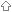Last update March 7, 2012

# Dwith Swig / Examples / Gi Na CTable of contents of this pageC++ classes for symbolic manipulationFeatures usedCode to be wrappedInterface fileRun SWIGExample applicationCompile and LinkOutput

###C++ classes for symbolic manipulationThe classes provided by GiNaC allow for symbolic expressions to be expressed and manipulated.

There are three classes provided in GiNaC which made are available in D using SWIG.

• symbol is an object initialised to a string. e.g.```symbol x = new symbol("x"); symbol y = new symbol("y");```

• ex is an expression involving one or more symbols. e.g.```ex exx = new ex(x); ex exy = new ex(y);```

• lst is a list of expressions. e.g.`lst l = new lst(exx,exy);`

An object of class lst can be used to initalise an expression from a string e.g.`ex e11 = new ex("x+y",l);`

where l provides a list of the symbols in the expression.

###Features usedThe features used here are the same as described in /Examples/Qdmath . The interface contains a lot of code to implement overloaded operators. The implementation of this in D is considerably easier than some other languages, such as Ruby and python.

###Code to be wrappedThis is contained in the header files for GiNaC. The current version uses GiNaC 1.1.4. This needs to be upadated to the current version.

###Interface fileThis is rather long so I will not put it here. It is getting more urgent to set up a location for distribution of these files to those who would like them. It was not a long task to adapt the interface file which I already used for Ruby.

###Run SWIG`swig -dmd -c++ ginacd.i`

The output is the following files

• ginacd.d
• classsymbol.d
• classex.d
• classlst.d
• ginacdPINVOKE.d
• ginacd_wrap.cxx

###Example applicationrunginacd.d```// Experiments with GiNaC for D.. // Imports conversion stuff from C import std.string; import std.intrinsic; import std.math; import classsymbol; int main(char[][] args) { printf("GiNaC for D\n"); symbol x = new symbol("x"); printf("x = %s\n",x.str()); symbol y = new symbol("y"); printf("y = %s\n",y.str()); ex exx = new ex(x); ex exy = new ex(y); lst l = new lst(exx,exy); printf("l = %s\n",l.str()); ex e0 = new ex(0); ex e1 = new ex(1); printf("e1 = %s\n",e1.str()); ex e11 = new ex("x+y",l); printf("e11 = %s\n",e11.str()); printf("e1 + e11 = %s\n",(e1+e11).str()); printf("e11 - e1 = %s\n",(e11-e1).str()); printf("e11 * e11 = %s\n",(e11*e11).str()); printf("e11 / e11 = %s\n",(e11/e11).str()); printf("x + y = %s\n",(x+y).str()); printf("e1 + y = %s\n",(e1+y).str()); printf("x + e1 = %s\n",(x+e1).str()); printf("1 + e11 = %s\n",(1+e11).str()); printf("1.5 + e11 = %s\n",(1.5+e11).str()); printf("2*x + 3*y = %s\n",(2*x+3*y).str()); ex es = 1 + 3*x/(x+y); printf("es = 1 + 3*x/(x+y) = %s\n",es.str()); printf("es.normal() = %s\n",es.normal().str()); printf("End of tests\n"); return 0; }```

###Compile and LinkLinux```dmd -c runginacd.d dmd -c ginacd.d dmd -c classsymbol.d dmd -c classex.d dmd -c classlst.d g++ -c ginacd_wrap.cxx g++ runginacd.o -orunginacd ginacd.o classsymbol.o classex.o classlst.o ginacd_wrap.o -lginac -lphobos -lpthread -lm```

Windows

GiNaC is not available for Windows.

###Output```GiNaC for D x = x y = y l = {x,y} e1 = 1 e11 = y+x e1 + e11 = 1+y+x e11 - e1 = -1+y+x e11 * e11 = (y+x)^2 e11 / e11 = 1 x + y = y+x e1 + y = 1+y x + e1 = 1+x 1 + e11 = 1+y+x 1.5 + e11 = 1.5+y+x 2*x + 3*y = 3*y+2*x es = 1 + 3*x/(x+y) = 1+3*(y+x)^(-1)*x es.normal() = (y+x)^(-1)*(y+4*x) End of tests```

| | | | | | | | | |

(date of last change: March 7, 2012 20:27 )